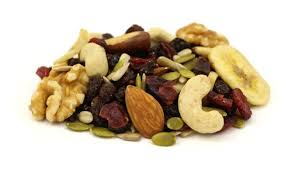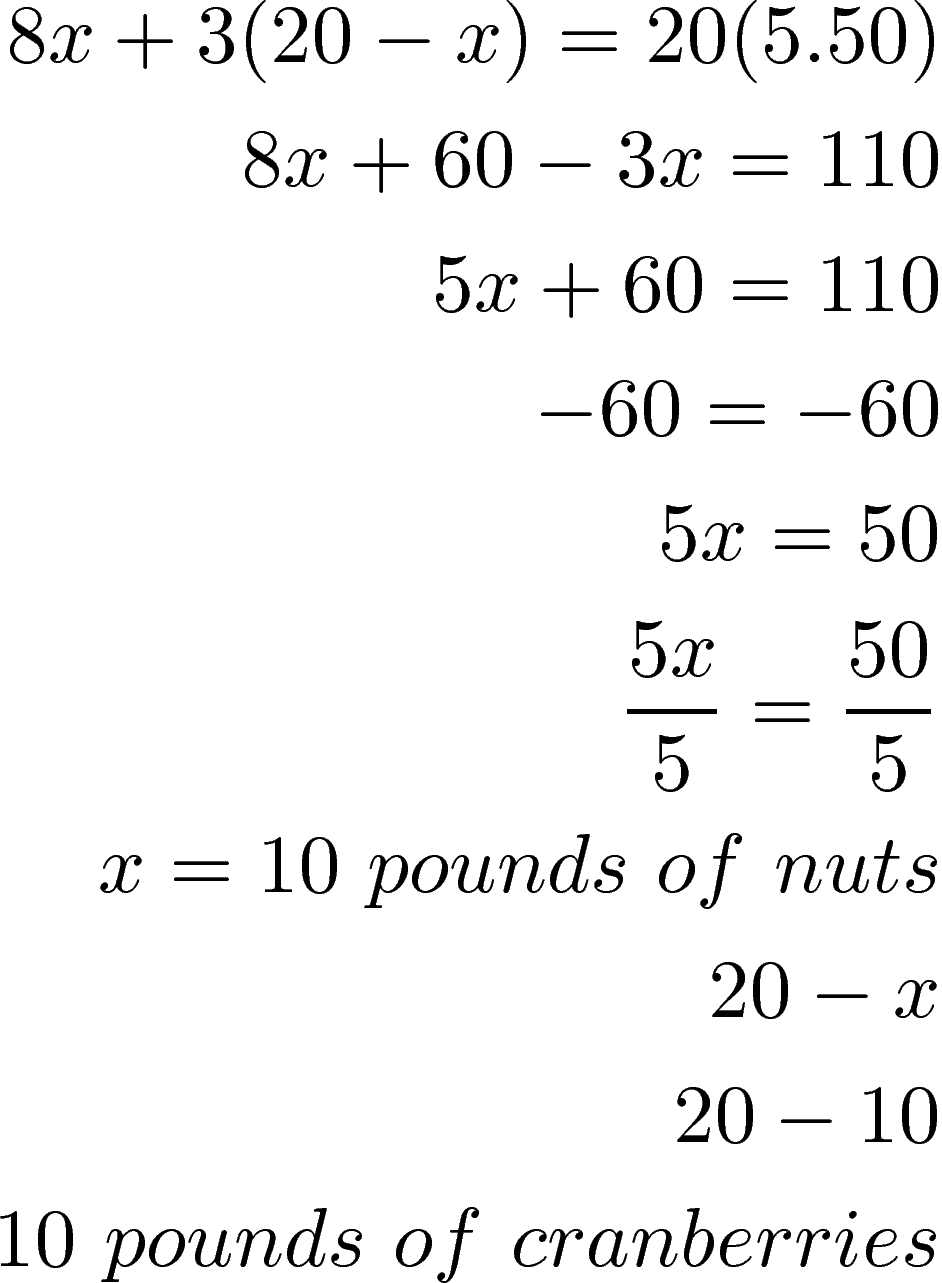# Algebraic Mixture Problems

There are many examples in the world in which you want to know the quantity of several different items that make up a whole. When such a situation arise it is an example of mixture problem.In this post, we will look at several examples of mixture problems. First, we need to look at the general equation for a mixture problem.

number * value = total value

The problems we will tackle will all involve some variation of the equation above. Below is our first example

Example 1

There are times when you want to figure out how many coins are needed to equal a certain dollar amount such as in the problem below

Tom has \$6.04 of pennies and nickels. The number of nickels is 4 more and 6 times the number of pennies. How many nickels and pennies does Tom have?

To have success with this problem we need to convert the information into a table to see what we know. The table is below.

Type Number * Value = Total Value
Pennies x .01 .01x
Nickels 6x+4 .05 .05(6x+4)

total 6.04

We can now solve our equation.We know that there are 18.83 pennies. To determine the number of nickels we put 18.83 into x and get the following.Almost 117 nickels

You can check if this works for yourself.

Example 2

For those of us who love to cook, mixture equations can be used for this as well below is an example.

Tom is mixing nuts and cranberries to make 20 pounds of trail mix. Nuts cost \$8.00 per pound and cranberries cost \$3.00 per pound. If Tom wants to his trail mix to cost \$5.50 per pound how many pounds of raisins and cranberries should he use?

Our information is in the table below. What is new is subtracting the number of pounds from x. Doing so will help us to determine the number of pounds of cranberries.

Type Number of Pounds* Price Per Pound = Total Value
nuts x 8 8x
Cranberries 20-x 3 3(20-x)
Trail Mix 20 5.5 20(5.50)

We can now solve our equation with the information in the table above.Once you solve for x you simply place this value into the equation. When you do this you see that we need ten pounds of nuts and berries to reach our target cost.

Conclusion

This post provided to practical examples of using algebra realistically. It is important to realize that understanding these basics concepts can be useful beyond the classroom.